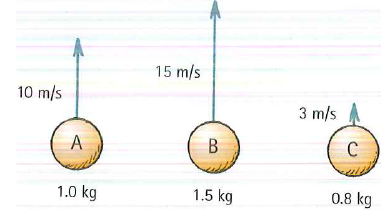# Problem: Three balls of different masses are thrown straight upward with initial speeds as indicateda. From fastest to slowest, rank the speeds of the balls 1s after being thrownb. From greatest to least, rank the accelerations of the balls 1s after being thrown (Or are the accelerations the same?)

###### FREE Expert Solution

Uniform accelerated motion (UAM) equations, a.k.a. "kinematics equations":

Remember, mass has no effect on the speed or acceleration of an object in free fall

Using the first UAM equation:

vf,A = 10 - 10 × 1 = 0.0 m/s

100% (445 ratings)###### Problem Details

Three balls of different masses are thrown straight upward with initial speeds as indicateda. From fastest to slowest, rank the speeds of the balls 1s after being thrown

b. From greatest to least, rank the accelerations of the balls 1s after being thrown (Or are the accelerations the same?)

Frequently Asked Questions

What scientific concept do you need to know in order to solve this problem?

Our tutors have indicated that to solve this problem you will need to apply the Vertical Motion and Free Fall concept. You can view video lessons to learn Vertical Motion and Free Fall. Or if you need more Vertical Motion and Free Fall practice, you can also practice Vertical Motion and Free Fall practice problems.

What professor is this problem relevant for?

Based on our data, we think this problem is relevant for Professor Solis' class at ASU.